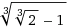# Surd Problem 2

Algebra Level 5$x$, $y$ and $z$ are rational numbers such that

$\sqrt{\sqrt{2}-1} = \sqrt{x}+\sqrt{y}+\sqrt{z}$

If $x+y+z$ can be expressed as $\dfrac{m}{n}$, where $m$ and $n$ are relatively prime positive integers,

find $m+n$.

×